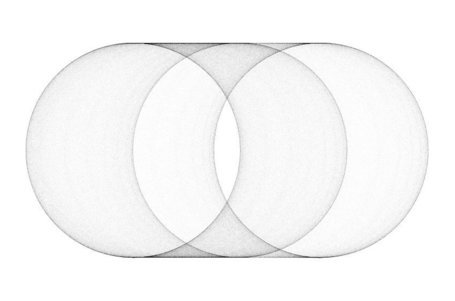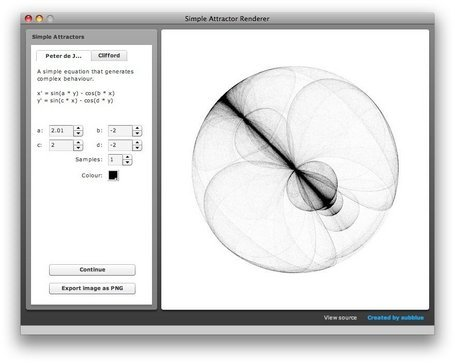Visit the new site

# Simple Attractors

Published on 26 May 2008

## This little experiment lets you visually explore two very simple attractor equations that generate very unexpected and aesthetically pleasing results.It was coded in Actionscript 3.0 and wrapped into an Adobe Air application so that you can export the rendered imaged as PNGs.

The two simple attractor equations are credited to Peter de Jong:

`x' = sin(a * y) - cos(b * x)y' = sin(c * x) - cos(d * y)`

And Cliff Pickover:

`x' = sin(a * y) + c * cos(a * x)y' = sin(b * x) + d * cos(b * y)`

Start with a random point x, y and plot a semi-transparent pixel at the calculated x', y' point. Plug the new x', y' values back into the equations to get the next point, and so on for a few hundred thousand iterations. Vary the a, b, c, d constants to give wildly different results.

View the gallery for more renderings.

Note: you will need the Adobe Air runtime installed first.Last updated: 5 October 2008